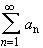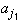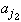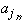#Interactive Real Analysis

Next | Previous | Glossary | Map

## 4.1. Series and Convergence

### Theorem 4.1.6: Absolute Convergence and Rearrangement

Letbe an absolutely convergent series. Then any rearrangement of terms in that series results in a new series that is also absolutely convergent to the same limit.

Letbe a conditionally convergent series. Then, for any real number c there is a rearrangement of the series such that the new resulting series will converge to c.

### Proof:

Supposeis absolutely convergent. Then the sequence
Sn = |a1| + |a2| + ... + | an|
converges. In particular, it is bounded, i.e. |Sn| < K for some number K. If we take any rearrangement of terms in the series and form a new sequence of partial sums:
Tn = || + || + ... ||
then Tn is again bounded by the same number K. But since all terms in the partial sum Tn are positive the sequence is monotone increasing. Therefore {Tn} is monotone increasing and bounded and must therefore converge.

It remains to show that the limit of the rearrangement is the same as the limit of the original series. That is left as an exercise.

Finally suppose the seriesconverges conditionally. Let's first collect a few facts:

• By the divergence test (which we will prove later) we know that the sequence of general terms an converges to zero.
• The series does not converge absolutely.
• Since the sequence of partial sums of absolute values is increasing it means that the series of absolute values must "converge" to positive infinity.
• There must be infinitely many positive terms among the aj. If we call them bj and collect them to form a series then that new series must "converge" to positive infinity (why?).
• There must be infinitely many negative terms among the aj. If we call them cj and collect them to form a series then that new series must "converge" to negative infinity (why?).
Now we can describe the idea of the proof, leaving the details as an exercise:
• Collect enough bj so that they add up to a number just bigger than c
• Add as many terms from cj as is necessary to make the resulting sum just less than c
• Add again terms from bj to be just bigger than c, then again terms from cj to be less than c, and so on.

Then you can show that the resulting arrangment of bj's and cj indeed forms a series that converges to c. As usual, the details of this proof are left as an exercise.Next | Previous | Glossary | Map